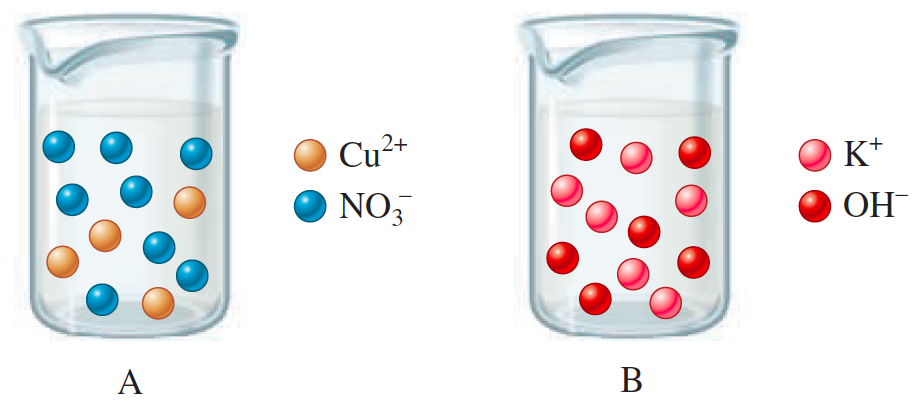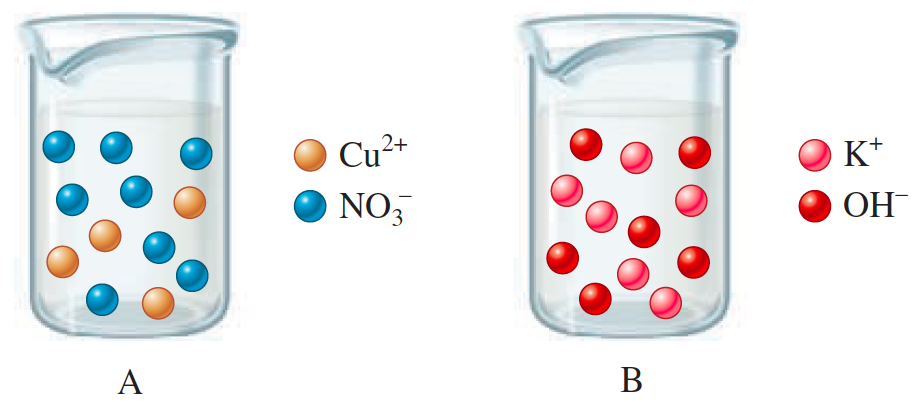# Problem: The drawings below represent aqueous solutions. Solution A is 2.00 L of a 2.00-M aqueous solution of copper(II) nitrate. Solution B is 2.00 L of a 3.00-M aqueous solution of potassium hydroxide.Draw a picture of the solution made by mixing solutions A and B together after the precipitation reaction takes place. Make sure this picture shows the correct relative volume compared to solutions A and B, and the correct relative number of ions, along with the correct relative amount of solid formed.

###### FREE Expert Solution

Cu(NO3)2 (aq)   +  2 KOH (aq)  →  Cu(OH)2(s)   +   2 KNO3 (aq)4 Cu(NO3)2 (aq)                          6 KOH

82% (265 ratings)###### Problem Details

The drawings below represent aqueous solutions. Solution A is 2.00 L of a 2.00-M aqueous solution of copper(II) nitrate. Solution B is 2.00 L of a 3.00-M aqueous solution of potassium hydroxide.Draw a picture of the solution made by mixing solutions A and B together after the precipitation reaction takes place. Make sure this picture shows the correct relative volume compared to solutions A and B, and the correct relative number of ions, along with the correct relative amount of solid formed.